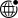Solutions
Overview of Silicon Photonics Circuit Design

Silicon photonics chips for optical communication systems, with increasing importance, scale integration technology is also supported by the process foundries, as electronic circuits using Spice simulator, in the silicon-based optoelectronic link design also has a corresponding pSim circuit simulator and design methods. Engineers use pSim circuit simulators as analysis and optimization tools to shorten design cycles, and simulation provides the opportunity to cost-effectively test ideas, optimize designs, and solve problems. Next in the circuit design and simulation approach, we first describe the application of each photonic device in an optical communication system, and then use the results of a compact model obtained from the physical electromagnetic and electrical solver to simulate a physically realistic silicon-based optoelectronic link. Simulation of silicon-based photonic circuitss requires a combination of system, circuit, and device simulations. System-based simulations require transient analysis or simulation of the waveforms of optical and electrical signals in the time domain. Device simulations, on the other hand, are based on physical solutions and usually require simulation of Maxwell's equations or drift diffusion and Poisson's equations. Above all, the same device can have different models in different domains. For example, a ring resonator can be modeled in the system/circuit domain using analytical equations or scattering matrices, while in the device domain it can be modeled using the full vector solution of Maxwell's equations. This series of articles focuses on the system/link level, where it is desired to obtain key performance metrics, such as eye diagrams, to understand which subcircuits and devices adversely affect the overall system performance, and also to define target specifications for key devices to achieve the desired performance (coupling coefficient, insertion loss, return loss, etc.).

You can follow this series of articles to build a circuit in pLogic and simulate the link through pSim, as shown below, both of which are modules of the full flow of the domestic EDA software PIC Studio optoelectronic fusion design.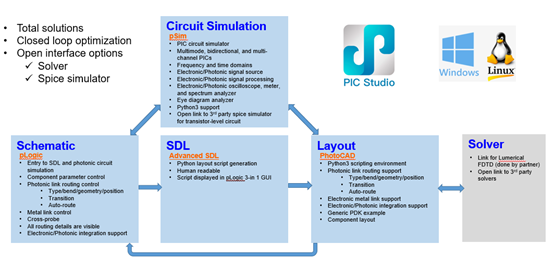Figure: PIC Studio Co-simulation Full Design Flow

Simulation and Analysis of Silicon Photonics Devices in Optical Communication Circuit

This series of articles introduces the performance simulation and analysis of silicon-based photonic devices in optical communication circuit applications, from theory to practice, the operating tool is the pSim simulator of Progressive Technology Company, which supports optoelectronic mixed signal generation and simulation, users can use pLogic visualization graphics to pull devices to form a link and set up the simulation, after the simulation you can watch the output waveform of the optoelectronic signal. Three link designs are introduced in the basic section serially.

n  Optical bandpass filter with micro-loop assistance.

n  Linearity and sensitivity analysis of photodetectors applied to optical receivers.

n  Characterization of micro-loop modulators applied to data center optical interconnects.

Optical Bandpass Filter with Micro-loop Assistance

The application of optical bandpass filters includes demultiplexing and add/drop functions in wavelength division multiplexing systems.

Circuit Function Description

A passive micro-loop-assisted MZI is built via pLogic as shown in the figure below. From the op_3 port of the optical network analyzer (ONA_1), the optical power is attenuated using a laser source emitted as a light source to an optical attenuator (ATT_1). the PSR is a composite device consisting of a polarization beam splitter and a polarization rotator. The PSR is then used to separate the light source into two ports. One port is connected to a terminal (TERM_2). The other port is connected to a straight waveguide (WGD_1). The micro-ring-assisted MZI (my_rmzi_1) is a compound device that requires a self-built device, introduced as a device connecting the left and right ends, and consists of eight micro-ring resonators. The laser source is injected from the op_0 port of my_rmzi_1. The terminal reflector (TERM_1) is connected to the op_1 port of MZI_1 and the reflectivity is set to 0.0001 to reflect the optical signal.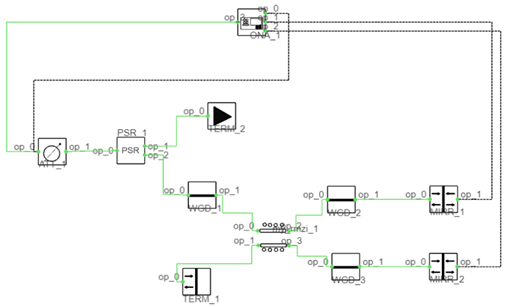Figure 2: Schematic of MZI with passive micro-loop assistance via pLogic

The laser source is then divided into two ports (op_2 and op_3 of my_rmzi_1). One port is connected to the waveguide (WGD_2) and the optical mirror (MIRR_1), and the other port is connected to the waveguide (WGD_3) and the optical mirror (MIRR_2). The reflectance of both optical mirrors is set to 0.0001. s11 set to 1 means that the phase difference between the reflected spectrum and the incident spectrum is π. After passing through the waveguide and the optical mirror, the two optical signals are connected to the op_1 port and the op_2 port of ONA_1 to measure the received signal. Finally, the op_0 port of ONA_1 is connected to the op_ 0 port in ATT_1 to detect the return loss signal.

Description of operating principle

The micro-loop-assisted MZI structure can be applied to band-pass filters. The frequency response is an important parameter of the bandpass filter. The function of frequency response can be expressed as: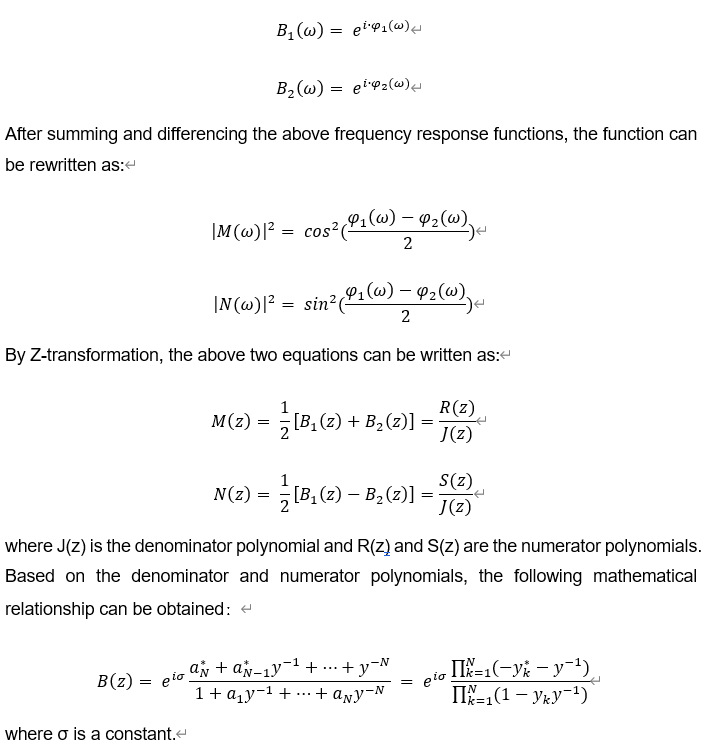Then, the internal connection of the passive micro-loop-assisted MZI link is built by pLogic, as shown in the following figure. The operation methods, parameters, and simulation settings of the relevant details of the custom devices are detailed in the "pSim-based design of silicon-based optoelectronic integrated links" tutorial, and will not be repeated here.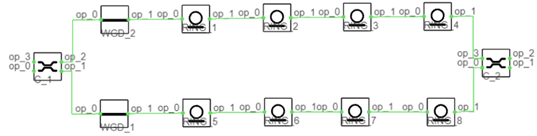Figure 3: Internal connection of a passive micro-loop-assisted MZI link

With the pSim simulation link, the simulation results of the passive micro-loop-assisted MZI link can be observed using the waveform viewer built into pSim.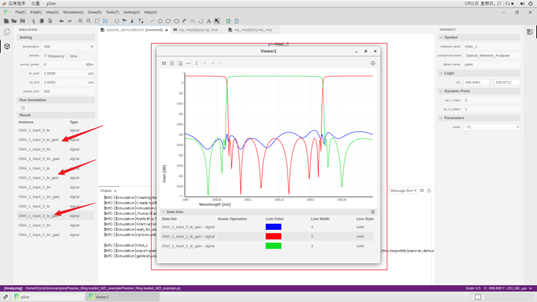Figure 4: Simulation results of the passive micro-loop-assisted MZI link obtained by pSim

The subsequent series of articles will introduce the linearity and sensitivity analysis of photodetectors applied to optical receivers and the characterization of micro-loop modulators applied to data center optical interconnects via pSim.